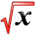# WorkThis article is part of the MathHelp Tutoring Wiki

Work= Force x Distance

Example: How much work is exerted to lift a 1.5 kg box from the floor onto a table that is 1.2 meters tall?

Solution:

Using acceleration due to gravity 9.8 m/s2, find Force.

Force= mass x acceleration = 1.5x9.8= 14.7 N

Using the equation W=Force x Distance, find work.

Work= 14.7 x 1.2 = 17.64 Joules

Hooke's Law: f(x)= kx where k is the spring constant and x is the amount the spring is stretched beyond its length at rest

Example: A force of 35 N is exerted to keep a spring stretched 5 cm beyond its natural length. How much work is required to stretch the spring 3 cm more?

Solution: We know from Hooke's Law that the force needed to maintain a spring stretched x metres beyond the length at rest is f(x)= kx.

5cm=0.05m So 35=0.05k

Therefore k= 35/0.05= 700

Now we have f(x)=700x

The work required to stretch 3 cm more becomes:

W= ∫0.080.05700xdx= 700 (x2/2) from 0.05 to 0.08=

```   * Back to Integral Calculus
```